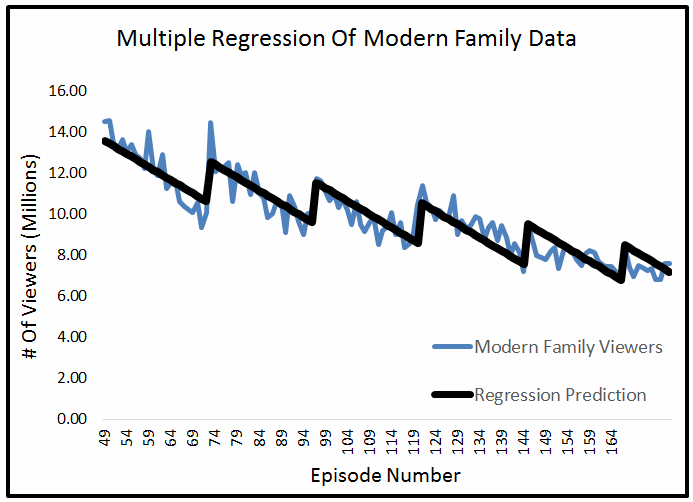## Liner Regression Examples

### Easy To Understand Examples Solved In Excel

This Excel file shows examples of implementing Linear Regression for a number of different problems.  The examples show things such as simple linear regression, correlation, and R squared.  Some of the more complicated examples show how to solve multiple linear regression as a series of simple linear regressions.

All of the examples are solved in Excel, and you can quickly change the input or the functions to get new results for different problems.

50% Complete

You're Almost there! Please complete this form and click the button below to gain instant access.• Progressively more difficult problems exploring different aspects of Linear Regression
• Examples on real world data, such as recreating Moore's Law and predicting the number of viewers on future episodes of television shows
• Examples with the goal of giving you an intuitive understanding of Linear Regression
• Solutions to complicated problems, such as how to do Multiple Regression as a series of simple linear regressions, which make it easy to understand
• All examples are set up to easily change the input, and see the effects